GET THE APP

Temperature Variation in Transient State of a Thermal Insulator and the Time Fractional Diffusion Equation

# Journal of Applied & Computational Mathematics

ISSN: 2168-9679

Open Access
Submit Manuscript arrow_forward arrow_forwardResearch Article - (2021) Volume 10, Issue 12

# Temperature Variation in Transient State of a Thermal Insulator and the Time Fractional Diffusion Equation

Rasolomampiandry G1*, Rakotoson R2 and Randimbindrainibe F3
*Correspondence: Rasolomampiandry G, Cognitive Sciences and Applications Research Laboratory, BP 1500, Ankatso - Antananarivo 101, Madagascar, Email:
1Cognitive Sciences and Applications Research Laboratory (LR - SCA), Madagascar
2Doctoral School in Engineering and Innovation Sciences and Techniques (ED - STII), Madagascar
3Ecole Supérieure Polytechnique Antananarivo (ESPA) - University of Antananarivo, BP 1500, Ankatso - Antananarivo 101, Madagascar

Citation: Rasolomampiandry G, Rakotoson R, Randimbindrainibe F. "Temperature Variation in Transient State of a Thermal Insulator and the Time Fractional Diffusion Equation." J Appl Computat Math 9 (2021): 497.
Copyright: © 2021 Rasolomampiandry G. This is an open-access article distributed under the terms of the Creative Commons Attribution License, which permits unrestricted use, distribution, and reproduction in any medium, provided the original author and source are credited.

## Abstract

This work consists in studying the adequacy of the experimental results and the theoretical results obtained from the time fractional diffusion equation on the variation of the temperature, in transient regime, during the heating of a thermal insulator. called "thermisorel".

#### Keywords

Fractional diffusion equation • Experimental results • Thermisorel

#### Introduction

In , a heat transfer study was performed on a sample of low density wood fiber board, called Thermisorel. This material is manufactured by STEICO Casteljaloux in France. Thermisorel is used in construction because they avoid heat loss in winter due to their low thermal conductivity, nearly 0.042 W m-1 K-1. It also protects the building from heat in summer due to their capacity high thermal storage.

Details of the study are described in . Only the data necessary for our problem mentioned above, which we will recall.

This work will be divided into three parts:

In section 2, we will perform a discretization of the time fractional diffusion equation. The third part consists in extracting all the data necessary to solve our problem. In particular the experimental results on the on the variation of the temperature, in transient mode, during the heating of this thermal insulator. In the next part, we will develop the fractional diffusion equation according to the data obtained in . Then we will do its numerical resolution, in order to be able to compare the results with the experimental data.In this study we used a 22.2 mm thick thermisorel board

#### Discretization of the fractional thermal diffusion equation

We will only consider the one-dimensional case, i.e. the temperature depends only on the real variable x and the time t. The time fractional thermal diffusion equation is written: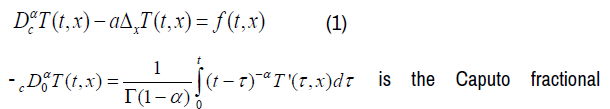derivative of order α,

where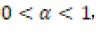of the real variable t, t>0 [2,3]

- ‘a ’is the diffusivity coefficient

T(t, x) is the temperature corresponding to the variables t and x

f (t, x) is the second member of equation (1) for the variables t and x

For our discretization: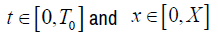On the segment [0,T0 ], we construct a finite sequence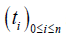such that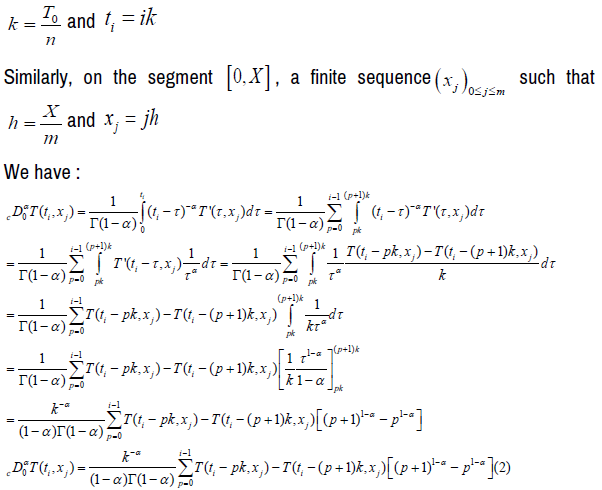This relation is valid for i ≠ 0

For the discretization of the Laplacian one will use the advanced decentered differentiation  and we have: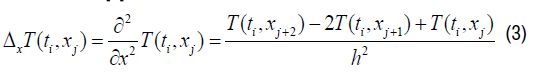Note: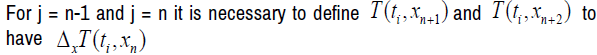The equation (1) has become: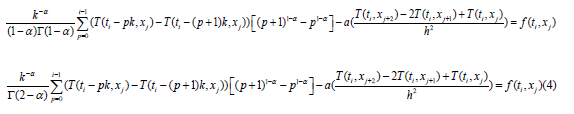Brief Description and Results of the Experiment

Experimentation

As already mentioned above, that in , a study on the transient heat transfer on thermal insulation was made. During the experiment, we took a sample of thermisorel plate of thickness E = 22.2mm. The sample was placed between the two plates of a flowmeter and the following temperature specifications were used:

The temperature of one plate should be kept at 15.4 °C and the other plate should raise the temperature from 15.4 to 24.7° C.

The heat flux between the samples and each plate was measured. The same initial temperature was measured (approximately 15.4°C). The temperature rise took several minutes and measurements were taken every 10 second until steady state was reached.

The heat flux measurements were carried out by the CSTB (Scientific and Technical Center for Building) in Grenoble. For the results of this experiment see 

The macroscopic properties of the thermisorel

Several properties of this material are mentioned in . In this paragraph, only the parameters necessary for the resolution of our problem we will recall: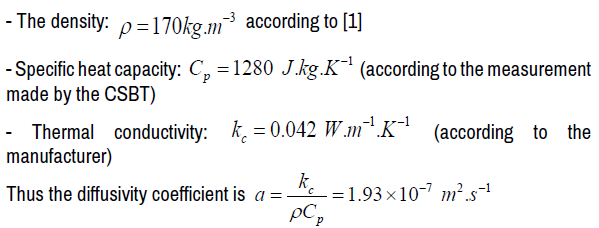Note:

According to , the heat transfer by convection and by radiation are negligible, so only the heat transfer by conduction is considered.

Experimental results obtained for the thermisorel

In , there are two results of the experiment which are given as a graphical representation. One represents the change in temperature and the other the flux, as a function of time, on both sides of the thermisorel sample.

From these graphs, we extracted the values of the temperature and those of the flux as a function of time, until the steady state was reached, for the hot side. We have the following (Tables 1 and 2)

Table 1: Table representation of the temperature variation of the hot face during the transient regime.

Time in second Température in °C
0 15
10 15
20 15.67
30 16.34
40 17.34
50 17.5
60 18
70 19
80 20
90 21
100 22.34
110 23.67
120 24.33
130 24.66

Table 2: Representation in table form of the variation of the flux of the hot face during the transient regime.

Time in second Flux in W .m-2
0 0
10 0
30 18.34
40 20
50 30
60 33.33
70 38.34
80 41 .67
90 46 .67
100 43.34
110 38.34
120 31.67
130 18 .37

Numerical resolution of the time fractional thermal diffusion equation

To study the temperature variation on the hot face of the thermisorel, it suffices to study an arbitrary point on this face. From this point, we will consider a straight line segment of length E = 22.2mm and which is perpendicular to the face. On which, we will define a time fractional thermal diffusion equation on segment, including the unknown is the temperature at time t at a point of abscissa x on this line segment, denoted T (t, x).

The equation of the problem

From (1) the equation takes the form: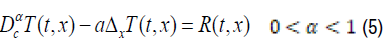The radiation effect and the convection effect are negligible, but the existence of the heat source must be considered so we can assume that the corresponding equation is with second member:

For the initial conditions we have:

The transient regime lasts 130 seconds, then t ∈[0,130]

The length of the line segment is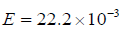meters, then x∈[0, E]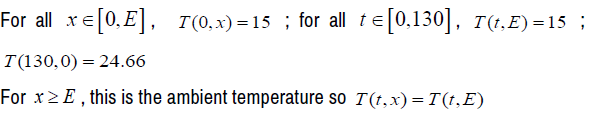The discretization gives us:

- On the segment [0,130] , we build a finite sequence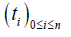such that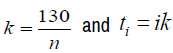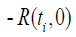is equal to the flux observed at the instant on the hot face (see table 2)

- Similarly, on the segment [0,E] , a finite sequence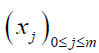such that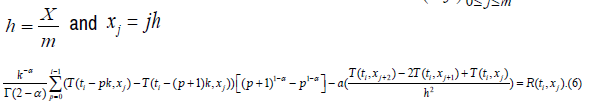As in the experiment, we will give the values T(t,0) for t ∈[0,130]

For that ,we will take h = E and k = 10 then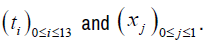And we write (6) by taking j = 0and 0 <i≤13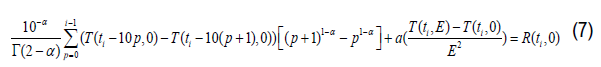Relation (7) is the discretization corresponding to the time fractional thermal diffusion equation, in accordance with the hypothesis of the experiment.

Numerical resolution

According to the results obtained in the previous paragraph, our problem turns to finding values of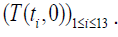This requires a system of 13 equations with 13 unknowns that we will build from (7), as follows:

We will write (7) for i = 1,....,13 :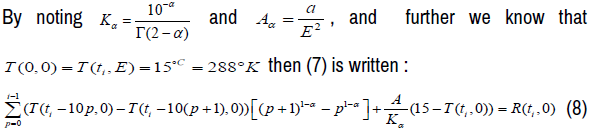From (8) We obtain a system of Thirteen equations with thirteen unknowns, whose unknowns are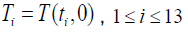as following :

The first equation is: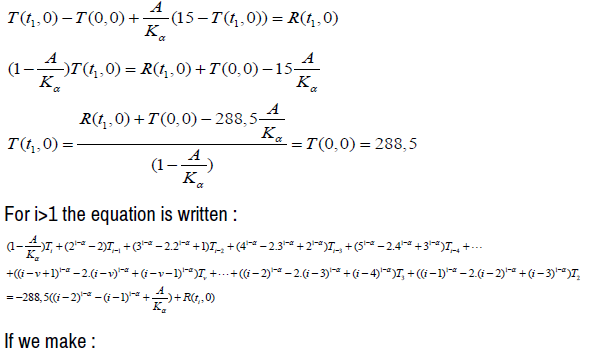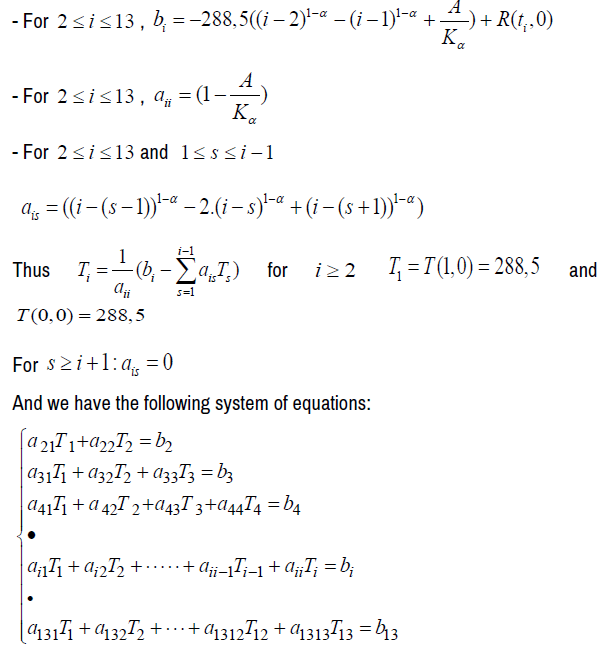Numerical resolution and results

With the hypotheses described previously, the method of Gauss and starting from the software MATLAB. We have the graph representing the experimental data and the theoretical results when α = 0.9. And according to the calculation under MATLAB, the difference between the values of the temperature resulting from the experimental method and the values obtained by simulation is 10.09% on average. This percentage is largely sufficient to confirm the adequacy of the experimental data with the theoretical values obtained, from the fractional diffusion equation with respect to time, when α = 0.9 (Figure 1&2).

Figure 1: Graphical representation of the temperature variation. If the time t varies between 0 and 1 and the position x between 0 and 2, when the order of the fractional derivative is 0.9.

Figure 2: Graphical representation of experimental data and theoretical values of temperature variations in transient regime of the hot face of the thermisorel as a function of time

#### Conclusion

From these results, we can say once again that we should not be content to use classical derivatives, in the studies of phenomena which require differential equations.

#### Journal Highlights

###### Citations: 1282

Journal of Applied & Computational Mathematics received 1282 citations as per Google Scholar report